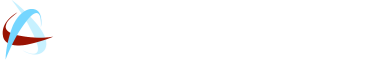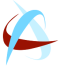# Georgia Sales Tax Auditor Factor

October 9, 2018Sales Tax

Georgia Sales Tax Auditor Factor

The sales tax audit factor can also be referred to as the average tax error and/or audit factor. Since most auditors refer to this number as the Factor, we will also call it the factor in this explanation.

The Factor is the most crucial number in the entire audit. If the audit is determined to have a high Factor, then, the liability will simply correspond with that number. A Factor of 8 was considered high until we just came across an audit recently where the audit Factor was 12. The difference is astronomical.

For each stratum, the average tax error for the sample is determined by summing all the errors in the stratum sample and dividing by the stratum sample size.

For example, 70 transactions from a stratum are examined. Three transactions are found to have tax errors. For one transaction, the tax error is an underpayment of 26 cents. The other two transactions also have under payments of .49 and .86. So the average sample error for the stratum is .26 + .49 + .86/70 = .023. Using this method, one can deduce that the sales tax is understated by .023. Or, one can say that the error rate in sales tax reported is .023.

However, in the real world, the audit figures will look more like this: A 36 month period will be examined – 2015, 2016, and 2017.. The stratum here is 36. Then, the auditor will select 3 months out of that period and sample those three months for error – let’s use January, May, and September of 2016. When the auditor finds that \$100 was unreported in January, \$200 in May, and \$300 in September, we use the following formula:

Step 1: \$100 + \$200 + \$300 = \$600

Step 2:  \$600/36 months (length of audit period) = 16.6 Factor

Therefore, the error rate and/or factor = 16.6; the Taxpayer is presumed to have underreported sales tax by 16.6.

Georgia Sales Tax Auditor Factor